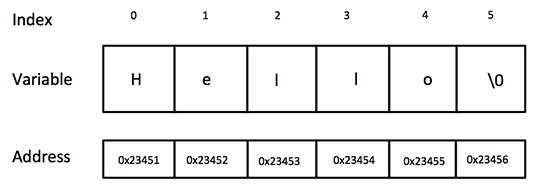C++

# C++ 字符串

## C++字符串

C++ 提供了以下两种类型的字符串表示形式：

• C 风格字符串
• C++ 引入的 string 类类型

## C 风格字符串

C?风格的字符串起源于 C?语言，并在 C++ 中继续得到支持。字符串实际上是使用 null 字符 ‘\0‘ 终止的一维字符数组。因此，一个以 null 结尾的字符串，包含了组成字符串的字符。

```char greeting = {‘H‘, ‘e‘, ‘l‘, ‘l‘, ‘o‘, ‘\0‘};
```

```char greeting[] = "Hello";
``````#include <iostream>

using namespace std;

int main ()
{
char greeting = {‘H‘, ‘e‘, ‘l‘, ‘l‘, ‘o‘, ‘\0‘};

cout << "Greeting message: ";
cout << greeting << endl;

return 0;
}
```

```Greeting message: Hello
```

C++ 中有大量的函数用来操作以 null 结尾的字符串：supports a wide range of functions that manipulate null-terminated strings:

1 strcpy(s1, s2);

2 strcat(s1, s2);

3 strlen(s1);

4 strcmp(s1, s2);

5 strchr(s1, ch);

6 strstr(s1, s2);

```#include <iostream>
#include <cstring>

using namespace std;

int main ()
{
char str1 = "Hello";
char str2 = "World";
char str3;
int  len ;

// 复制 str1 到 str3
strcpy( str3, str1);
cout << "strcpy( str3, str1) : " << str3 << endl;

// 连接 str1 和 str2
strcat( str1, str2);
cout << "strcat( str1, str2): " << str1 << endl;

// 连接后，str1 的总长度
len = strlen(str1);
cout << "strlen(str1) : " << len << endl;

return 0;
}
```

```strcpy( str3, str1) : Hello
strcat( str1, str2): HelloWorld
strlen(str1) : 10
```

## C++ 中的 String 类

C++ 标准库提供了 string 类类型，支持上述所有的操作，另外还增加了其他更多的功能。我们将学习 C++ 标准库中的这个类，现在让我们先来看看下面这个实例：

```#include <iostream>
#include <string>

using namespace std;

int main ()
{
string str1 = "Hello";
string str2 = "World";
string str3;
int  len ;

// 复制 str1 到 str3
str3 = str1;
cout << "str3 : " << str3 << endl;

// 连接 str1 和 str2
str3 = str1 + str2;
cout << "str1 + str2 : " << str3 << endl;

// 连接后，str3 的总长度
len = str3.size();
cout << "str3.size() :  " << len << endl;

return 0;
}
```

```str3 : Hello
str1 + str2 : HelloWorld
str3.size() :  10
```